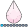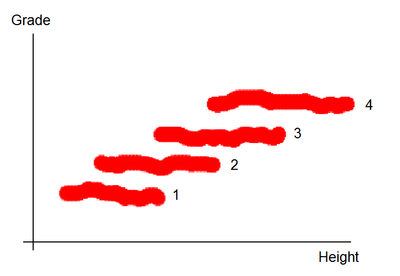NKormanik
Barite | Level 11

## See distributions of student height by grade level

Would someone please steer me in the right direction for creating the following:In the example above, there are four grade levels, and height generally overlaps to a degree.

Ideally the plot should show a distribution, clustering toward the center of each grade, and outliers at either end.

Thanks very much!

Nicholas Kormanik

1 ACCEPTED SOLUTION

Accepted Solutions

## Re: See distributions of student height by grade level

I like draycut's solution because it seems closest to the image that you posted. However, be aware that box plots show a schematic representation of the distribution, and jittering breaks down when you get to thousands of observations. For larger samples, look at comparative histograms, which scale to larger samples.

7 REPLIES 7

## Re: See distributions of student height by grade level

My goto place for anything graph related is this site:

http://blogs.sas.com/content/graphicallyspeaking/

There are thousands of examples there.

## Re: See distributions of student height by grade level

Follow this example and use HBOX instead of VBOX in PROC SGPLOT.

http://blogs.sas.com/content/graphicallyspeaking/2017/06/16/scatter-mean-value/

Plot sample data if you want a code answer 🙂

## Re: See distributions of student height by grade level

Hi,

You can try proc boxplot for this type of analysis. It will compare distribution of height at each grade. Also highlights outliers and skewness.

## Re: See distributions of student height by grade level

I like draycut's solution because it seems closest to the image that you posted. However, be aware that box plots show a schematic representation of the distribution, and jittering breaks down when you get to thousands of observations. For larger samples, look at comparative histograms, which scale to larger samples.NKormanik
Barite | Level 11

## Re: See distributions of student height by grade level

Rick, I think the first plot from your article will work well for my purpose.

Code:

``````proc univariate data=sas_1.divisions_20905;
class Rank;
var i_20905;
histogram i_20905 / nrows=7 odstitle="i_20905";
ods select histogram;
run;``````

Two follow-up questions:

1. Could we overlay some statistical information within the plot, such as percentile numbers?

2. Output plot files need to be appropriately named.  In the case above, "i_20905".

Thanks so much for your help.  As well as to the others here.NKormanik
Barite | Level 11

## Re: See distributions of student height by grade level

The following seems to work out pretty well.  Thanks again for everyones help.

``````ods graphics on / reset=index imagename="20905";

proc univariate data=sas_1.divisions_20905;
class rank (order=data);
var i_20905;
histogram i_20905 / nrows=7 odstitle="20905";
inset nobs max p95 p75 mean p50 p25 p5 min / format=6.1 pos=nw;
ods select histogram;
run;
``````

## Re: See distributions of student height by grade level

Doesn't show "outliers" but something like this perhaps:

```data example;
do i=1 to 1000;